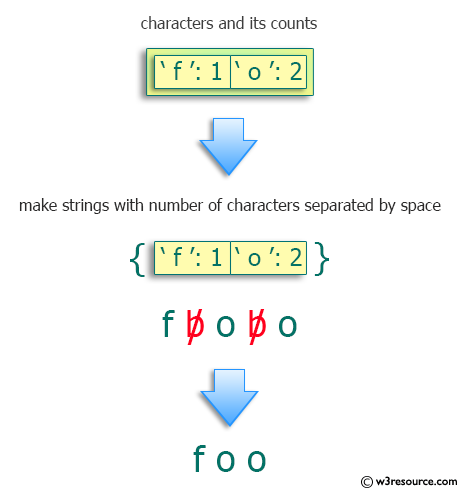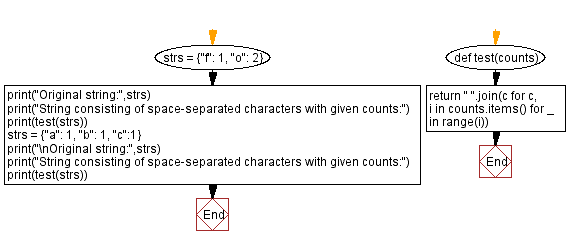﻿ Python: Find a string consisting of space-separated characters with given counts - w3resource# Python: Find a string consisting of space-separated characters with given counts

## Python Programming Puzzles: Exercise-74 with Solution

Write a Python program to find a string consisting of space-separated characters with given counts.

```Input: {'f': 1, 'o': 2}
Output:
f o o

Input: {'a': 1, 'b': 1, 'c': 1}
Output:
a b c
```

Pictorial Presentation:Sample Solution:

Python Code:

``````#License: https://bit.ly/3oLErEI

def test(counts):
return " ".join(c for c, i in counts.items() for _ in range(i))
strs = {"f": 1, "o": 2}
print("Original string:",strs)
print("String consisting of space-separated characters with given counts:")
print(test(strs))
strs = {"a": 1, "b": 1, "c":1}
print("\nOriginal string:",strs)
print("String consisting of space-separated characters with given counts:")
print(test(strs))
``````

Sample Output:

```Original string: {'f': 1, 'o': 2}
String consisting of space-separated characters with given counts:
f o o

Original string: {'a': 1, 'b': 1, 'c': 1}
String consisting of space-separated characters with given counts:
a b c
```

Flowchart:## Visualize Python code execution:

The following tool visualize what the computer is doing step-by-step as it executes the said program:

Python Code Editor :

Have another way to solve this solution? Contribute your code (and comments) through Disqus.

What is the difficulty level of this exercise?

Test your Programming skills with w3resource's quiz.

﻿

## Python: Tips of the Day

Clamps num within the inclusive range specified by the boundary values x and y:

Example:

```def tips_clamp_num(num,x,y):
return max(min(num, max(x, y)), min(x, y))
print(tips_clamp_num(2, 4, 6))
print(tips_clamp_num(1, -1, -6))
```

Output:

```4
-1
```